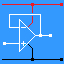RANDOM PAGE

SITE SEARCH

LOG
IN

HELP

# Wave Terminology

This is the AQA version closing after June 2019. Visit the the version for Eduqas instead.

a

## Wave Technical Terms

b

### Sine Wave

• This image shows a sine wave.
• It has a smooth regular shape.
• It is special because this signal contains a single frequency.
• Other wave shapes contain multiple frequencies.c

### Cycle

• One complete wave including one peak and one trough.
d

### Wavelength

• The distance between one peak and the next. Any point on the wave could be used but peaks are convenient.
e

### Frequency

The number of cycles per second measured in Hertz (Hz).

• Frequency = 1 / Period
f

### Period

The time for one complete cycle to pass measured in seconds.

• Period = 1 / Frequency
g

### Peak

• The highest point on the wave.
h

### Trough

• The lowest point on the wave.
i

### Displacement

• The distance the medium has moved from its mean position.
j

### Amplitude

• This is the peak (of maximum) displacement. The size of the wave measured from the centre line usually in Volts.

k

### Polarisation

• The orientation of the electric Field. (Usually Horizontal, Vertical or Circular).
l

### Calculating The Wavelength

• Speed of Light = Frequency * Wavelength
• C = F λ
• λ = C / F
m

### Calculating The Frequency

• Speed of Light = Frequency * Wavelength
• C = F λ
• F = C / λ
• C = 3x108 ms-1

reviseOmatic V3     Contacts, ©, Cookies, Data Protection and Disclaimers Hosted at linode.com, London